Next: Generating the Edit Up: Overview and Preliminaries Previous: Edit Scripts

## A Cost Model for Edit Scripts

Given two trees, in general there are many edit scripts that transform one tree to the other. Even when an edit script must conform to a given matching, there may be many correct scripts. (Recall that we defined the concept of an edit script conforming to a matching in Section 3.) For example, the following edit script, when applied to the initial tree in Example 3.1, produces the same final tree as that produced by the edit script in the example: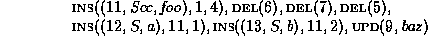Intuitively, this edit script does more work than necessary, and is thus an undesirable representation of the delta between the trees. To formalize this idea, we introduce the cost of an edit script.

We first define the costs of edit operations and then use these costs to define the cost of edit scripts. The cost of an edit operation depends on the type of operation and the nodes involved in the operation. Let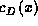,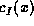, and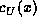denote respectively the cost of deleting, inserting, and updating node x, and let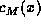denote the cost of moving the subtree rooted at node x. In general, these costs may depend on the label and the value of x, as well as its position in the tree. In this paper, we adopt a simple cost model where deleting and inserting a node, as well as moving a subtree, are considered to be unit cost operations. That is,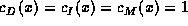for all x.

Now consider the costof updating the value of a node x. We assume that this cost is given by a function, compare, that evaluates how different x's old value v is from its new value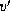. This compare function takes two nodes as arguments and returns a number in the range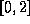. Although the nature of the compare function is arbitrary, it should be consistent with the costs of the other edit operations in the following sense: Suppose x is moved, and its value v is updated so that v is very similar to. Then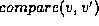should be less than 1, so that the cost of moving and updating x is less than the cost of deleting x and replacing it with a new node with value. If v andare very different, we would rather have the edit script contain a delete/insert pair, so the update cost should be greater than 1. Finally, the cost of an edit script is the sum of the costs of its individual operations.Next: Generating the Edit Up: Overview and Preliminaries Previous: Edit Scripts

Sudarshan S. Chawathe
Wed Jun 19 08:22:34 PDT 1996